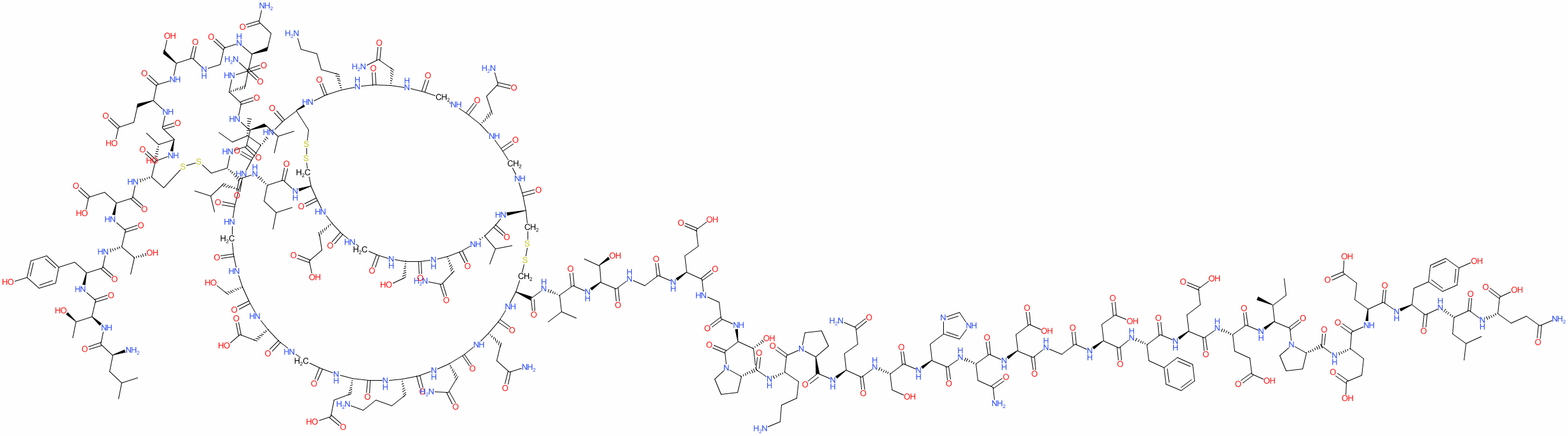#### GtoPdb Ligand ID: 6469

Synonyms: 1-L-leucine-2-L-threonine-63-desulfohirudin | Refludan®
lepirudin is an approved drug (FDA (1998))
Comment: Discontinued (not withdrawn). The drug is a recombinant form of hirudin, the salivary anticoagulant found in leeches (see UniProt P01050), but has two amino acid modifications; substitution of Leu1 for Ile and no sulfate group on Tyr63. The PubChem entry linked to above represents the chemical structure of Lepirudin.View more information in the IUPHAR Pharmacology Education Project: lepirudin

2D StructureSMILES / InChI / InChIKeyCanonical SMILES NCCCC[C@@H](C(=O)N1CCC[C@H]1C(=O)N[C@H](C(=O)N[C@H](C(=O)N[C@H](C(=O)N[C@H](C(=O)N[C@H](C(=O)NCC(=O)N[C@H](C(=O)N[C@H](C(=O)N[C@H](C(=O)N[C@H](C(=O)N[C@H](C(=O)N1CCC[C@H]1C(=O)N[C@H](C(=O)N[C@H](C(=O)N[C@H](C(=O)N[C@H](C(=O)N[C@H](C(=O)O)CCC(=O)N)CC(C)C)Cc1ccc(cc1)O)CCC(=O)O)CCC(=O)O)[C@H](CC)C)CCC(=O)O)CCC(=O)O)Cc1ccccc1)CC(=O)O)CC(=O)O)CC(=O)N)Cc1nc[nH]c1)CO)CCC(=O)N)NC(=O)[C@@H]1CCCN1C(=O)[C@H]([C@H](O)C)NC(=O)CNC(=O)[C@@H](NC(=O)CNC(=O)[C@H]([C@H](O)C)NC(=O)[C@H](C(C)C)NC(=O)[C@@H]1CSSC[C@@H]2NC(=O)[C@@H](NC(=O)[C@H](CC(=O)N)NC(=O)[C@H](CO)NC(=O)CNC(=O)[C@H](CCC(=O)O)NC(=O)[C@H](CSSC[C@H](NC(=O)[C@@H](NC(=O)[C@@H](NC(=O)CNC(=O)[C@@H](NC(=O)CNC2=O)CCC(=O)N)CC(=O)N)CCCCN)C(=O)N[C@@H]([C@H](CC)C)C(=O)N[C@H](C(=O)NCC(=O)N[C@H](C(=O)N[C@H](C(=O)NCC(=O)N[C@H](C(=O)N[C@H](C(=O)N[C@H](C(=O)N[C@H](C(=O)N1)CCC(=O)N)CC(=O)N)CCCCN)CCC(=O)O)CC(=O)O)CO)CC(C)C)NC(=O)[C@@H](NC(=O)[C@@H]1CSSC[C@H](NC(=O)[C@@H](NC(=O)[C@H]([C@H](O)C)NC(=O)[C@@H](NC(=O)[C@H]([C@H](O)C)NC(=O)[C@H](CC(C)C)N)Cc2ccc(cc2)O)CC(=O)O)C(=O)N[C@@H]([C@H](O)C)C(=O)N[C@@H](CCC(=O)O)C(=O)N[C@H](C(=O)NCC(=O)N[C@H](C(=O)N[C@H](C(=O)N[C@H](C(=O)N1)CC(C)C)CC(=O)N)CCC(=O)N)CO)CC(C)C)C(C)C)CCC(=O)O Isomeric SMILES NCCCC[C@@H](C(=O)N1CCC[C@H]1C(=O)N[C@H](C(=O)N[C@H](C(=O)N[C@H](C(=O)N[C@H](C(=O)N[C@H](C(=O)NCC(=O)N[C@H](C(=O)N[C@H](C(=O)N[C@H](C(=O)N[C@H](C(=O)N[C@H](C(=O)N1CCC[C@H]1C(=O)N[C@H](C(=O)N[C@H](C(=O)N[C@H](C(=O)N[C@H](C(=O)N[C@H](C(=O)O)CCC(=O)N)CC(C)C)Cc1ccc(cc1)O)CCC(=O)O)CCC(=O)O)[C@H](CC)C)CCC(=O)O)CCC(=O)O)Cc1ccccc1)CC(=O)O)CC(=O)O)CC(=O)N)Cc1nc[nH]c1)CO)CCC(=O)N)NC(=O)[C@@H]1CCCN1C(=O)[C@H]([C@H](O)C)NC(=O)CNC(=O)[C@@H](NC(=O)CNC(=O)[C@H]([C@H](O)C)NC(=O)[C@H](C(C)C)NC(=O)[C@@H]1CSSC[C@@H]2NC(=O)[C@@H](NC(=O)[C@H](CC(=O)N)NC(=O)[C@H](CO)NC(=O)CNC(=O)[C@H](CCC(=O)O)NC(=O)[C@H](CSSC[C@H](NC(=O)[C@@H](NC(=O)[C@@H](NC(=O)CNC(=O)[C@@H](NC(=O)CNC2=O)CCC(=O)N)CC(=O)N)CCCCN)C(=O)N[C@@H]([C@H](CC)C)C(=O)N[C@H](C(=O)NCC(=O)N[C@H](C(=O)N[C@H](C(=O)NCC(=O)N[C@H](C(=O)N[C@H](C(=O)N[C@H](C(=O)N[C@H](C(=O)N1)CCC(=O)N)CC(=O)N)CCCCN)CCC(=O)O)CC(=O)O)CO)CC(C)C)NC(=O)[C@@H](NC(=O)[C@@H]1CSSC[C@H](NC(=O)[C@@H](NC(=O)[C@H]([C@H](O)C)NC(=O)[C@@H](NC(=O)[C@H]([C@H](O)C)NC(=O)[C@H](CC(C)C)N)Cc2ccc(cc2)O)CC(=O)O)C(=O)N[C@@H]([C@H](O)C)C(=O)N[C@@H](CCC(=O)O)C(=O)N[C@H](C(=O)NCC(=O)N[C@H](C(=O)N[C@H](C(=O)N[C@H](C(=O)N1)CC(C)C)CC(=O)N)CCC(=O)N)CO)CC(C)C)C(C)C)CCC(=O)O InChI Key FIBJDTSHOUXTKV-BRHMIFOHSA-N
 ClassificationCompound class Peptide or derivative Approved drug? Yes (FDA (1998))
 International Nonproprietary NamesINN number INN 7355 lepirudin
 Synonyms1-L-leucine-2-L-threonine-63-desulfohirudin | Refludan®
 Database LinksSpecialist databases Reactome DrugR-ALL-9603268 Reactome ReactionR-HSA-9603302 Other databases CAS Registry No. 138068-37-8 (source: PubChem) ChEMBL Ligand CHEMBL1201666 DrugBank Ligand DB00001 GtoPdb PubChem SID 178103083 PubChem CID 16132441 Search Google for chemical match using the InChIKey FIBJDTSHOUXTKV-BRHMIFOHSA-N Search Google for chemicals with the same backbone FIBJDTSHOUXTKV Search PubMed clinical trials lepirudin Search PubMed titles lepirudin Search PubMed titles/abstracts lepirudin Search UniChem for chemical match using the InChIKey FIBJDTSHOUXTKV-BRHMIFOHSA-N Search UniChem for chemicals with the same backbone FIBJDTSHOUXTKV Wikipedia Lepirudin